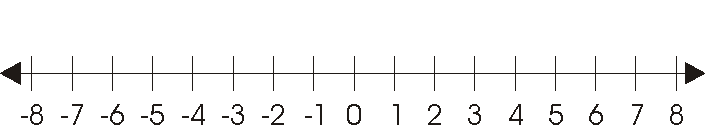Absolute Value

The absolute value of a number is the distance from zero to that number on a number line. Using math symbols absoulute value is written as: |number|. Examples are: |12| which is 12, or |3| which is 3, or |-28| which is 28. Another way to think about absolute value is that you just make the number non-negative. Zero and any number larger than zero are non-negative (not negative) so they stay the same. Any number that is less than zero is negative so you have to change the sign on the number to make it non-negative.